Query Optimization in DBMS

We have seen so far how a query can be processed based on indexes and joins, and how they can be transformed into relational expressions. The query optimizer uses these two techniques to determine which process or expression to consider for evaluating the query.

There are two methods of query optimization.

1. Cost based Optimization (Physical)

This is based on the cost of the query. The query can use different paths based on indexes, constraints, sorting methods etc. This method mainly uses the statistics like record size, number of records, number of records per block, number of blocks, table size, whether whole table fits in a block, organization of tables, uniqueness of column values, size of columns etc.

Suppose, we have series of table joined in a query.

T1 ∞ T2 ∞ T3 ∞ T4∞ T5 ∞ T6

For above query we can have any order of evaluation. We can start taking any two tables in any order and start evaluating the query. Ideally, we can have join combinations in (2(n-1))! / (n-1)! ways. For example, suppose we have 5 tables involved in join, then we can have 8! / 4! = 1680 combinations. But when query optimizer runs, it does not evaluate in all these ways always. It uses Dynamic Programming where it generates the costs for join orders of any combination of tables. It is calculated and generated only once. This least cost for all the table combination is then stored in the database and is used for future use. i.e.; say we have a set of tables, T = { T1 , T2 , T3 .. Tn}, then it generates least cost combination for all the tables and stores it.

• Dynamic Programming
Structure of DBMS

As we learnt above, the least cost for the joins of any combination of table is generated here. These values are stored in the database and when those tables are used in the query, this combination is selected for evaluating the query.

While generating the cost, it follows below steps :

Suppose we have set of tables, T = {T1 , T2 , T3 .. Tn}, in a DB. It picks the first table, and computes cost for joining with rest of the tables in set T.  It calculates cost for each of the tables and then chooses the best cost. It continues doing the same with rest of the tables in set T. It will generate 2n – 1 cases and it selects the lowest cost and stores it. When a query uses those tables, it checks for the costs here and that combination is used to evaluate the query. This is called dynamic programming.

In this method, time required to find optimized query is in the order of 3n, where n is the number of tables. Suppose we have 5 tables, then time required in 35 = 243, which is lesser than finding all the combination of tables and then deciding the best combination (1680). Also, the space required for computing and storing the cost is also less and is in the order of 2n. In above example, it is 25 = 32.

• Left Deep Trees

This is another method of determining the cost of the joins. Here, the tables and joins are represented in the form of trees. The joins always form the root of the tree and table is kept at the right side of the root. LHS of the root always point to the next join. Hence it gets deeper and deeper on LHS. Hence it is called as left deep tree.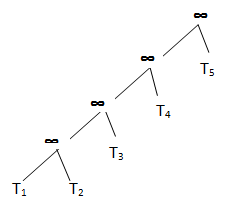Database Management System

Here instead of calculating the best join cost for set of tables, best join cost for joining with each table is calculated. In this method, time required to find optimized query is in the order of n2n, where n is the number of tables. Suppose we have 5 tables, then time required in 5*25 =160, which is lesser than dynamic programming. Also, the space required for computing storing the cost is also less and is in the order of 2n. In above example, it is 25 = 32, same as dynamic programming.

• Interesting Sort Orders

This method is an enhancement to dynamic programming. Here, while calculating the best join order costs, it also considers the sorted tables. It assumes, calculating the join orders on sorted tables would be efficient. i.e.; suppose we have unsorted tables T1 , T2 , T3 .. Tn and we have join on these tables.

(T1 ∞T2)∞ T3 ∞… ∞ Tn

This method uses hash join or merge join method to calculate the cost. Hash Join will simply join the tables. We get sorted output in merge join method, but it is costlier than hash join. Even though merge join is costlier at this stage, when it moves to join with third table, the join will have less effort to sort the tables. This is because first table is the sorted result of first two tables. Hence it will reduce the total cost of the query.

But the number of tables involved in the join would be relatively less and this cost/space difference will be hardly noticeable.

Tables in DBMS

All these cost based optimizations are expensive and are suitable for large number of data. There is another method of optimization called heuristic optimization, which is better compared to cost based optimization.

2. Heuristic Optimization (Logical)

This method is also known as rule based optimization. This is based on the equivalence rule on relational expressions; hence the number of combination of queries get reduces here. Hence the cost of the query too reduces.

This method creates relational tree for the given query based on the equivalence rules. These equivalence rules by providing an alternative way of writing and evaluating the query, gives the better path to evaluate the query. This rule need not be true in all cases. It needs to be examined after applying those rules. The most important set of rules followed in this method is listed below:

• Perform all the selection operation as early as possible in the query. This should be first and foremost set of actions on the tables in the query. By performing the selection operation, we can reduce the number of records involved in the query, rather than using the whole tables throughout the query.

Suppose we have a query to retrieve the students with age 18 and studying in class DESIGN_01. We can get all the student details from  STUDENT table, and class details from CLASS table. We can write this query in two different ways.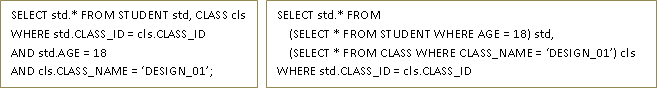Here both the queries will return same result. But when we observe them closely we can see that first query will join the two tables first and then applies the filters. That means, it traverses whole table to join, hence the number of records involved is more. But he second query, applies the filters on each table first. This reduces the number of records on each table (in class table, the number of record reduces to one in this case!). Then it joins these intermediary tables. Hence the cost in this case is comparatively less.

Views in DBMS

Instead of writing query the optimizer creates relational algebra and tree for above case.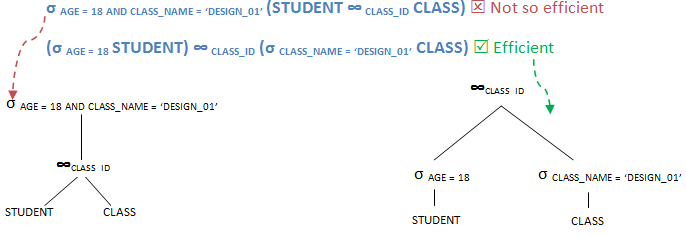• Perform all the projection as early as possible in the query. This is similar to selection but will reduce the number of columns in the query.

Suppose for example, we have to select only student name, address and class name of students with age 18 from STUDENT and CLASS tables.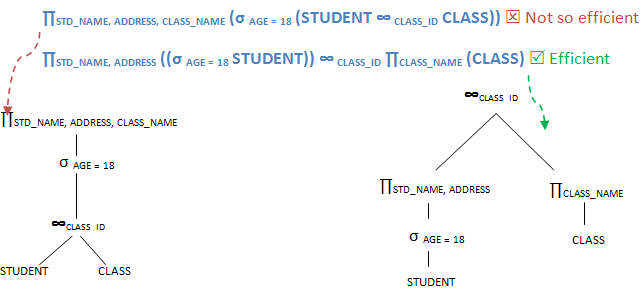Here again, both the queries look alike, results alike. But when we compare the number of records and attributes involved at each stage, second query uses less records and hence more efficient.

• Next step is to perform most restrictive joins and selection operations. When we say most restrictive joins and selection means, select those set of tables and views which will result in comparatively less number of records.  Any query will have better performance when tables with few records are joined. Hence throughout heuristic method of optimization, the rules are formed to get less number of records at each stage, so that query performance is better. So is the case here too.

Suppose we have STUDENT, CLASS and TEACHER tables. Any student can attend only one class in an academic year and only one teacher takes a class. But a class can have more than 50 students. Now we have to retrieve STUDENT_NAME, ADDRESS, AGE, CLASS_NAME and TEACHER_NAME of each student in a school.

∏STD_NAME, ADDRESS, AGE, CLASS_NAME, TEACHER_NAME ((STUDENT ∞ CLASS_ID CLASS)∞ TECH_IDTEACHER)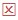Not So efficient

∏STD_NAME, ADDRESS, AGE, CLASS_NAME, TEACHER_NAME (STUDENT ∞ CLASS_ID (CLASS∞ TECH_IDTEACHER))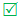Efficient